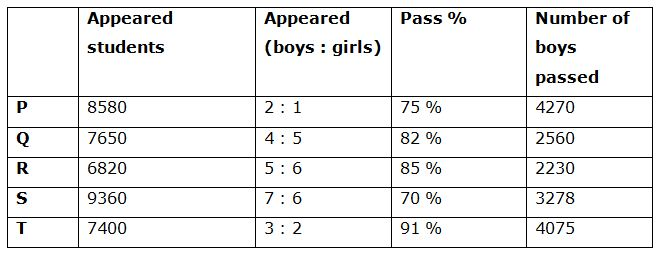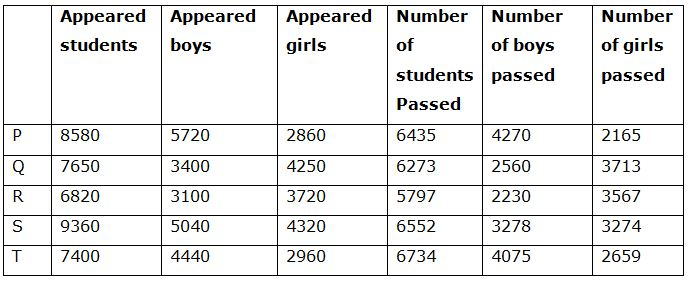# IBPS PO Prelims Quantitative Aptitude Questions 2019 (Day-7)

Dear Aspirants, Our IBPS Guide team is providing new series of Quantitative Aptitude Questions for IBPS PO Prelims 2019 so the aspirants can practice it on a daily basis. These questions are framed by our skilled experts after understanding your needs thoroughly. Aspirants can practice these new series questions daily to familiarize with the exact exam pattern and make your preparation effective.

Check here for IBPS PO Prelims Mock Test 2019

##### Check here for IBPS PO  Mock Test 2019
[WpProQuiz 6989]

1) If the numerator of a fraction is increased by 80% and the denominator of the fraction is increased by 120%, the resultant fraction becomes 5/11. Then what is the original fraction?

a) 6/7

b) 2/11

c) 3/14

d) 5/9

e) None of these

2) A, B and C together have Rs. 33250. If  3/7th of A’s amount is equal to 2/3rd of B’s amount and 1/4th of  B’s amount is equal to 5/8th of C’s amount, then how much amount does A have?

a) Rs. 15250

b) Rs. 16750

c) Rs. 19800

d) Rs. 17500

e) None of these

3) A, B and C started a business by investing an amount of Rs. 45000, Rs. 65000 and Rs. 70000 respectively. After 3 months, A invested Rs. 15000 more and B withdraw Rs. 5000. And after 4 months, C withdraw Rs. 15000. If the total profit at the end of the year is Rs. 114405, then find the share of A?

a) Rs. 35505

b) Rs. 32960

c) Rs. 28730

d) Rs. 26525

e) None of these

4) A 90 litres of mixture of milk and water contains milk and water in the ratio of 3: 2. How much water should be added to the mixture to get 45 % milk on it?

a) 26 litres

b) 30 litres

c) 22 litres

d) 18 litres

e) None of these

5) A boat can travel 55 km downstream in 66 min. The ratio of the speed of the boat in still water to the speed of the stream is 4: 1. How much time will the boat take to cover 72 km upstream?

a) 2 hours 48 mins

b) 3 hours 12 mins

c) 2 hours 24 mins

d) 3 hours 28 mins

e) None of these

Directions (6 – 10): Study the following information carefully and answer the given questions.

Following table shows the total number of students appeared for the examination from different cities and the ratio of appeared boys to girls among them and the percentage of students passed in the examination and number of boys passed in the examination.6) Find the average number of girls appeared for the examination from all the given cities together

a) 3156

b) 3480

c) 3622

d) 3378

e) None of these

7) The total number of girls passed in the city Q and R together is approximately what percentage of total number of girls passed in the city S and T together?

a) 156 %

b) 112 %

c) 145 %

d) 123 %

e) 137 %

8) Find the difference between the total number of girls appeared for the examination to that of total number of boys appeared for the examination from all the given cities together?

a) 4520

b) 3590

c) 2470

d) 5350

e) None of these

9) Find the ratio between the total number of girls passed from the city P and S together to that of total number of boys passed from the same city?

a) 1813: 2516

b) 1134: 1785

c) 1542: 2379

d) 2156: 2893

e) None of these

10) Total number of students appeared for the examination from city R and S together is approximately what percentage more/less than the total number of girls appeared for the examination from all the given cities together?

a) 15 % more

b) 27 % more

c) 11 % less

d) 39 % less

e) 8 % more

Direction (1-5) :

The original fraction be (x/y)

[X*(180/100)]/[Y*(220/100)] = 5/11

9x/11y = 5/11

x/y = 5/9

A + B + C = Rs. 33250

(3/7)* A = (2/3)* B

(A/B) = (2/3) * (7/3) = 14/9

(1/4)* B = (5/8)* C

(B/C) = (5/8) * (4/1) = 5/2

The ratio of A, B and C = 70: 45: 18

133’s = 33250

1’s = 250

70’s = Rs. 17500

The share of A, B and C,

= > [45000*3 + 60000*9]: [65000*3 + 60000*9]: [70000*7 + 55000*5]

= > 675000: 735000: 765000

= > 45: 49: 51

Total profit = Rs. 114405

145’s = 114405

1’s = 789

The share of A = 789*45 = Rs. 35505

Total mixture of milk and water = 90 litres

5’s = 90

1’s = 18

Total milk = 54 litres, water = 36 litres

According to the question,

54/(36 + x) = (45/55)

54/(36 + x) = 9/11

66 = 36 + x

X = 30

30 litres of water should be added to the mixture.

Speed of downstream = D/T = 55/(66/60) = 55*(60/66) = 50 km/hr

The ratio of the speed of the boat in still water to the speed of the stream

= > 4: 1 (4x, x)

5x = 50

X = 10

Speed of upstream = 4x – x = 3x = 30 km/hr

Distance = 752 km

Time = D/S = 72/30 = 2 2/5 hr = 2 hours 24 mins

Directions (6 – 10):The total number of girls appeared for the examination from all the given cities together

= > 2860 + 4250 + 3720 + 4320 + 2960

= > 18110

Required average = 18110/5 = 3622

The total number of girls passed in the city Q and R together

= > 3713 + 3567 = 7280

The total number of girls passed in the city S and T together

= > 3274 + 2659 = 5933

Required % = (7280/5933)*100 = 122.70 % = 123 %

The total number of girls appeared for the examination from all the given cities together

= > 2860 + 4250 + 3720 + 4320 + 2960

= > 18110

The total number of boys appeared for the examination from all the given cities together

= > 5720 + 3400 + 3100 + 5040 + 4440

= > 21700

Required difference = 21700 – 18110 = 3590

The total number of girls passed from the city P and S together

= > 2165 + 3274 = 5439

The total number of boys passed from the city P and S together

= > 4270 + 3278 = 7548

Required ratio = 5439: 7548 = 1813: 2516

Total number of students appeared for the examination from city R and S together

= > 6820 + 9360 = 16180

Total number of girls appeared for the examination from all the given cities together

= > 2860 + 4250 + 3720 + 4320 + 2960 = 18110

Required % = [(18110 – 16180)/18110]*100 = 10.65 % = 11 % less